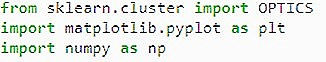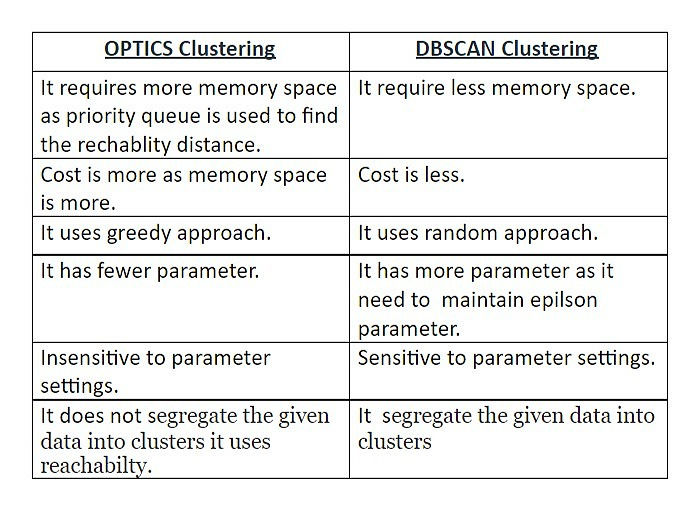top of page
Search

# OPTICS CLUSTERING

Updated: Aug 6, 2021

Author :-Tanya Juneja

Optics clustering or Ordering points to identify the clustering structure is an algorithm for finding density based clusters.## OPTICS has two Parameters:

• ε that describes the maximum distance or radiusMinPts which is number of

• points required to form a cluster.

It is inspires from DBSCAN algorithm. In DBSCAN we have we have 3 types of data points core point, noise or outlier and border point. We have added two more concepts into OPTICS.

• Core Distance: It is the minimum value of radius required to classify a given point as a core point.

• Reachability Distance: It is defined with respect to another data point q. The Reachability distance between a point p and q is the maximum of the Core Distance of p and the Euclidean Distance(or some other distance metric) between p and q.FIG:1

The Time complexity is O(n^2). OPTICS processes each point once, and it performs one of the neighborhood query during processing. As spatial index which grants a neighborhood query in runtime, an overall runtime of is obtained. The authors of the original OPTICS paper report an actual constant with slowdown factor of 1.6 as compared to DBSCAN. This may heavily influence the cost of the algorithm, as value is too large it increase the cost of a neighborhood query to linear complexity.

While choosing (larger than the maximum distance in the data set) is possible, but leads to quadratic complexity, since every neighborhood query returns the full data set. Even when no spatial index is available, this comes at additional cost in managing the heap. Therefore, should be chosen appropriately for the data set.

## Algorithm for OPTICS:

```OPTICS(DB, eps, MinPts)

#Repeating the process for all points in the database
for each point pt of DB

#Initializing the reachability distance of the selected point
pt.reachable_dist = UNDEFINED
for each unprocessed point pt of DB

#Getting the neighbours of the selected point
#according to the definitions of epsilon and
#minPts in DBSCAN
Nbrs = getNbrs(pt, eps)

mark pt as processed
output pt to the ordered list

#Checking if the selected point is not noise
if (core_dist(pt, eps, Minpts) != UNDEFINED)

#Initializing a priority queue to get the closest data  point
#in terms of Reachability distance
Seeds = empty priority queue

#Calling the update function
update(Nbrs, pt, Seeds, eps, Minpts)

#Repeating the process for the next closest point
for each next q in Seeds
Nbrs' = getNbrs(q, eps)
mark q as processed
output q to the ordered list
if (core_dist(q, eps, Minpts) != UNDEFINED)
update(Nbrs', q, Seeds, eps, Minpts)```

## Function in Python:-

`class sklearn.cluster.OPTICS(*, min_samples=5, max_eps=inf, metric='minkowski', p=2, metric_params=None,cluster_method='xi',eps=None,xi=0.05, predecessor_correction=True, min_cluster_size=None, algorithm='auto', leaf_size=30, n_jobs=None)`

## Parameters:

```min_samplesint > 1 or float between 0 and 1, default=5
The number of samples in a neighborhood for a point to be considered as a core point.

max_epsfloat, default=np.inf
The maximum distance between two samples in the neighborhood of the other.

metricstr or callable, default=’minkowski’
Metric to use for distance computation```

## Valid Values for Metric are:

• from scikit-learn: [‘cityblock’, ‘cosine’, ‘euclidean’, ‘l1’, ‘l2’, ‘manhattan’}

• from scipy.spatial.distance: [‘braycurtis’, ‘canberra’, ‘chebyshev’, ‘correlation’, ‘dice’, ‘hamming’, ‘jaccard’, ‘kulsinski’, ‘mahalanobis’, ‘minkowski’, ‘rogerstanimoto’, ‘russellrao’, ‘seuclidean’, ‘sokalmichener’, ‘sokalsneath’, ‘sqeuclidean’, ‘yule’]

• pint, default=2

• Parameter for the Minkowski metric from pairwise_distances. When p = 1, this is equivalent to using manhattan_distance (l1), and euclidean_distance (l2) for p = 2. For arbitrary p, minkowski_distance (l_p) is used.

• metric_paramsdict, default=None

• Additional keyword arguments for the metric function.

• cluster_methodstr, default=’xi’

• The extraction method used to extract clusters using the calculated reachability and ordering. Possible values are “xi” and “dbscan”.

• epsfloat, default=None

• The maximum distance between two samples for one to be considered as in the neighborhood of the other. By default it assumes the same value as max_eps. Used only when cluster_method='dbscan'.

• xifloat between 0 and 1, default=0.05

• Determines the minimum steepness on the reachability plot that constitutes a cluster boundary. For example, an upwards point in the reachability plot is defined by the ratio from one point to its successor being at most 1-xi. Used only when cluster_method='xi'

• predecessor_correctionbool, default=True

• Correct clusters according to the predecessors calculated by OPTICS . This parameter has minimal effect on most datasets. Used only when cluster_method='xi'.

• min_cluster_sizeint > 1 or float between 0 and 1, default=None

• Minimum number of samples in an OPTICS cluster, expressed as an absolute number or a fraction of the number of samples (rounded to be at least 2). If None, the value of min_samples is used instead. Used only when cluster_method='xi'.

• algorithm{‘auto’, ‘ball_tree’, ‘kd_tree’, ‘brute’}, default=’auto’

## Algorithm Used to Find the Nearest Neighbors:

• ‘ball_tree’ will use BallTree

• ‘kd_tree’ will use KDTree

• ‘brute’ will use a brute-force search.

• ‘auto’ will attempt to decide the most appropriate algorithm based on the values passed to fit method. (default)

• leaf_sizeint, default=30

• Leaf size passed to BallTree or KDTree.

• n_jobsint, default=None

• The number of parallel jobs to run for neighbors search.

## Steps for Optical Clustering using Python:

Step 1: Importing the required libraries:

Sklearn is used for forming clusters like optics,dbscan,etc.Matplotlib is used for creating graphs.Numpy for artimetic ,random generation and etc.One can load data using csv file or other file. For better understanding of clustering here data is randomly gemerated.Step 3: Preprocessing the Data:

Suppose there is data that is unnecessary for your clustering process you can remove them and normalize the data.It can be done by using normalize() and transform() from sklearn library.

Step 4: Building the Clustering Model:

Optics() method from sklearn is used.Step 5: Storing the results of the training:

The result is stored in label.Step 6: Visualizing the results using rechability graph and optics clustering:## Visualizing of Graph:-## OPTICS Clustering v/s DBSCAN Clustering:-## Code:-

```from sklearn.cluster import OPTICS
import matplotlib.pyplot as plt
import numpy as np

# Generate random sample data

np.random.seed(0)
n_points_per_cluster = 250

C1 = [-4, -1] + .8 * np.random.randn(n_points_per_cluster, 2)
C2 = [5, -1] + .1 * np.random.randn(n_points_per_cluster, 2)
C3 = [6, -2] + .2 * np.random.randn(n_points_per_cluster, 2)
C4 = [-7, 3] + .3 * np.random.randn(n_points_per_cluster, 2)
C5 = [6, -2] + 1.6 * np.random.randn(n_points_per_cluster, 2)
C6 = [4, 6] + 2 * np.random.randn(n_points_per_cluster, 2)
C7 = [-5, -8] + 2 * np.random.randn(n_points_per_cluster, 2)
X = np.vstack((C1, C2, C3, C4, C5, C6,C7))

clust = OPTICS(min_samples=50, xi=.05, min_cluster_size=.05)

# Run the fit
clust.fit(X)

#storing the results in label
space = np.arange(len(X))
reachability = clust.reachability_[clust.ordering_]
labels = clust.labels_[clust.ordering_]

# Reachability plot
colors = ['g.', 'r.', 'b.', 'y.', 'c.']
for klass, color in zip(range(0, 6), colors):
Xk = space[labels == klass]
Rk = reachability[labels == klass]
plt.plot(Xk, Rk, color, alpha=0.3)
plt.plot(space[labels == -1], reachability[labels == -1], 'k.', alpha=0.3)
plt.plot(space, np.full_like(space, 2., dtype=float), 'k-', alpha=0.5)
plt.plot(space, np.full_like(space, 0.5, dtype=float), 'k-.', alpha=0.5)
plt.ylabel('Reachability (epsilon distance)')
plt.title('Reachability Plot')
# OPTICS
colors = ['k.','g.', 'r.', 'b.', 'y*', 'w.']
for klass, color in zip(range(0, 6), colors):
Xk = X[clust.labels_ == klass]
plt.plot(Xk[:, 0], Xk[:, 1], color, alpha=0.3)
plt.plot(X[clust.labels_ == -1, 0], X[clust.labels_ == -1, 1], 'k+', alpha=0.1)
plt.title('OPTICS Clustering')
plt.show()```